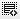## Question on Pandas adding String0

I have a database of 3000 rows and 100 columns which i have pulled in from the excel, I am trying to create a new column by adding string together which i want to create an index to merge databases.

ie DF["New column'}= DF["Column A"]+DF ["Column B"]. This is working for rows where we have string values present in both columns , when there is string value missing for only one row the new column is coming as blank for that row rather than taking the other cell.

5= 2+3

___= 2+___0

I believe what you are looking for here is:

df['col3'] = df[['col1', 'col2']].apply(lambda x: ''.join(x))

Or

df['col3'] = df[['col1', 'col2']].apply(lambda x: ''.join([str (I) for I in x]))

Click on thisicon to add code snippet.